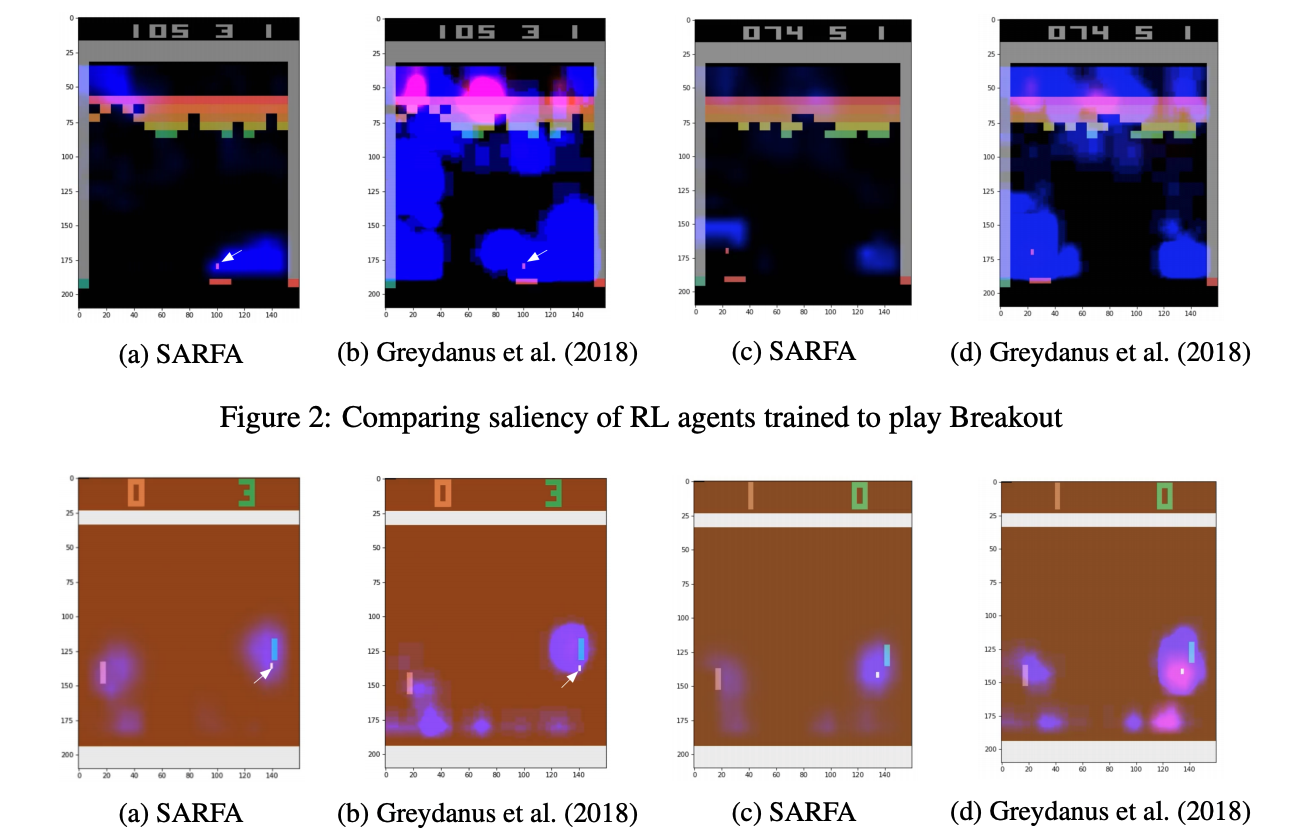# Explain Your Move: Understanding Agent Actions Using Specific and Relevant Feature Attribution¶

link

## SPECIFIC AND RELEVANT FEATURE ATTRIBUTION¶

Properties We define two desired properties of an accurate saliency map for policy-based agents:

1. Specificity: Saliency $S[f]$ should focus on the effect of the perturbation specifically on the action being explained, $\hat{a}$, i.e. it should be high if perturbing the $f^{\text {th }}$ feature of the state reduces the relative expected reward of the selected action. Stated another way, $S[f]$ should be high if $Q(s, \hat{a})-Q\left(s^{\prime}, \hat{a}\right)$ is substantially higher than $Q(s, a)-Q\left(s^{\prime}, a\right), a \neq \hat{a} .$ For instance, in figure 1, removing pieces such as the white queen impact all actions uniformly $\left(Q(s, a)-Q\left(s^{\prime}, a\right)\right.$ is roughly equal for all actions). Therefore, such pieces should not be salient for explaining $\hat{a}$. On the other hand, removing pieces such as the white knight on a4 specifically impacts the move $(\hat{a}=\mathrm{Bb} 6)$ we are trying to explain $\left(Q(s, B b 6)-Q\left(s^{\prime}, B b 6\right) \gg Q(s, a)-Q\left(s^{\prime}, a\right)\right.$ for other actions $a$ ). Therefore, such pieces should be salient for $\hat{a}$.
2. Relevance: Since the $Q$ -values represent the expected returns, two states $s$ and $s^{\prime}$ can have substantially different $Q$ -values for all actions, i.e. may be higher for $s^{\prime}$ for all actions if $s^{\prime}$ is a better state. Saliency map for a specific action $\hat{a}$ in $s$ should thus ignore such differences, i.e. $s^{\prime}$ should contribute to the saliency only if its effects are relevant to $\hat{a}$. In other words, $S[f]$ should be low if perturbing the $f^{\text {th }}$ feature of the state alters the expected rewards of actions other than $\hat{a}$. For instance, in Figure $1,$ removing the black pawn on c6 increases the expected reward of other actions (in this case, Bb4). However, it does not effect the expected reward of the action to be explained (Bb6). Therefore, the pawn is not salient for explaining the move. In general, such features that are irrelevant to $\hat{a}$ should not be salient.